Next: Distance Matrix Methods Up: Introduction Previous: Introduction

#### Parsimony

The parsimony methods usually count the number of amino acid or nucleotide substitutions in a weighted or unweighted manner. They take a multiple sequence alignment (MSA) as input and minimize the number of changes to explain the corresponding evolutionary tree. The construction of an optimal MSA, which is needed as input, is also NP complete . In addition, many algorithms for calculating MSAs need an evolutionary tree as input, which makes the problem circular.

Definition 1.2   Given is a set of sequences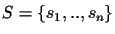withwhere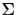is a finite alphabet. A Multiple Sequence Alignment (MSA) consists of a set of sequences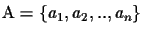with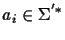where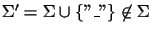.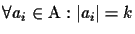. The sequence obtained from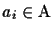by removing all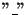gap characters is equal to si.

Chantal Korostensky
1999-07-14